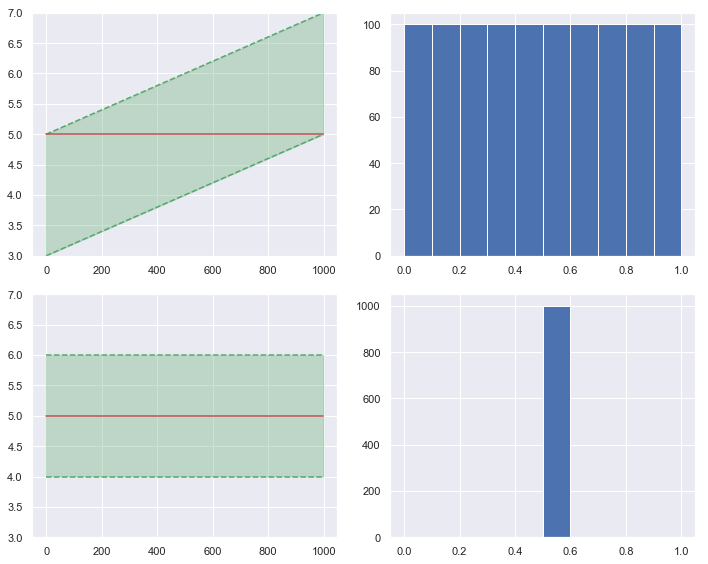## 1. 概率积分变换（Probability Integral Transform，PIT）

PIT 的优势之一是便于可视化。最简单的做法是画直方图。$\cup$ 形的直方图意味着预测的分布过于集中；$\cap$ 形的直方图意味着预测的分布过于分散；明显不对称的直方图则意味着预测的分布整体偏离真实值。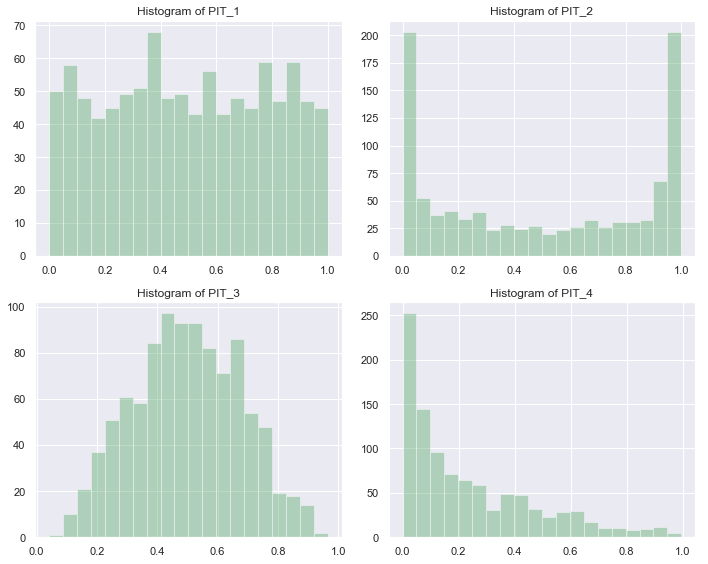PIT 还可以用 P-P 图来展示。简单地说，就是画出 PIT 的 CDF 与标准均匀分布的 CDF 的关系图。如果预测准确，得到的应该是一条直线。反 sigmoid 曲线意味着预测的分布过于集中；sigmoid 曲线意味着预测的分布过于分散；其它曲线则意味着预测的分布可能已经整体偏离真实值了。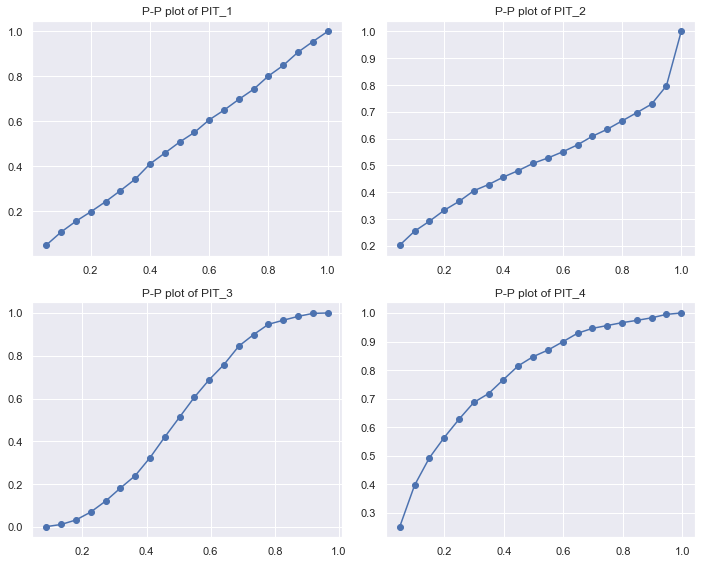## 2. 数值评分规则

### 2.1 连续概率排位分数（Continuous Ranked Probability Score，CRPS）

CRPS 是在概率预测领域使用最广泛的准确度指标之一。它的定义如下：

$CRPS(F^f, F^o) = \int_{-\infty}^{+\infty}\left[F^f(x)-F^o(x)\right]^2\mathrm dx \tag 1$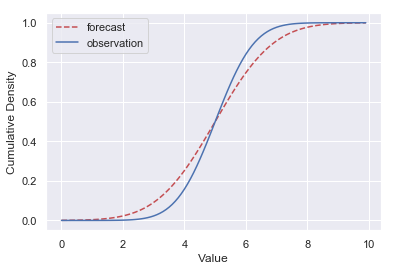\begin{aligned} CRPS(F, \xi) &=\frac 1n\sum_{i=1}^ncrps(F_i, \xi_i)\\ &= \frac 1n\sum_{i=1}^n\int_{-\infty}^{+\infty}\left[F_i(x)-\varepsilon(x-\xi_i)\right]^2\mathrm dx \end{aligned} \tag 2

$\varepsilon(t)= \begin{cases} 0, \qquad t<0\\ 1, \qquad t\geq0 \end{cases} \tag 3$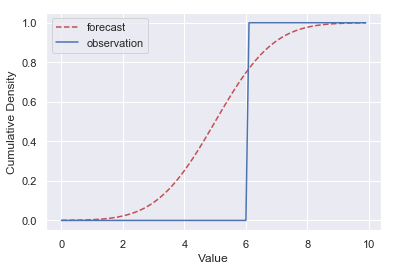### 2.2 交叉熵（Cross Entropy）和对数分数（Logarithmic Score）

$H(p, q) = \mathbb E_p[-\log_2q] \tag 4$

$p$$q$ 是离散的，则

$H(p, q) = -\sum_xp(x)\log_2q(x) \tag 5$

$H = -\frac1n\sum_{i=1}^n\log_2q(\xi_i) \tag 6$

$LogS(F, \xi) = -\frac1n\sum_{i=1}^n\log_2f_i(\xi_i) \tag 7$

### 2.3 Brier Score

Brier Score 通常用于分类问题中，其定义为

$BS = \frac1n\sum_{t=1}^n\sum_{i=1}^r(f_{ti}-o_{ti})^2 \tag 8$

## 3. 需要注意的问题